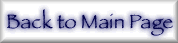## 8. Choice of Interpolation Formalism

The "convergence" with improved integration precision is partially due to inadequacy of interpolation methods rather than convergence to the value predicted by the independent particle approximation. The code of Cromer and Liberman  "interpolates" over ln(f″) using a quadratic least-squares fit. This smooths precision-dependent noise but also obscures significant local or higher-order structure. The 5 energies used are not well spaced for interpolation to other f″ values, whether for integration or otherwise. Reference  assumes a cubic form for the lnf″-lnE functional relation and uses the Aitken interpolation method; this combination generates non-monotonic and spurious f″ values near to or far above the edge. Reference  used a linear interpolation on log-log scales for E < 1 keV and noted significant errors for cubic spline interpolation at higher energies (for Z = 24, 42, 44, 67-69, 75, 76, 85).

Rational function interpolation is well able to model simple cubic terms (on the log-log scale) but is quite inappropriate for extended or oscillating structure. The latter would commonly produce a pole in the resulting fit near to the real plane and therefore generate large errors. Most investigators have (therefore) relied on polynomial interpolation on the log-log scale.

At energies far above the edge, the form f″(E) = f2(E) is expected to become approximately cubic, or linear in lnf″(ln E). With the photoabsorption data of Refs. [17-20], higher accuracy would follow from correct evaluation of higher order terms but data points are unequally spaced, so divergences easily follow extrapolation or interpolation. This may be limited by extrapolating or interpolating at either end of the energy range with a linear log-log form, while allowing intermediate values to be affected by higher order contributions. Interpolating functions may further be limited to symmetric forms about the region interpolated (i.e., to linear, cubic or quintic log-log interpolation using n = 2, 4, or 6 data points). Given a sufficiently fine grid, interpolation becomes insensitive to the form used. However, the database discussed herein lacks such a fine grid, drawing attention to the appropriate forms of interpolation. This is illustrated in Fig. 4 for medium energy attenuation by uranium, where the asterisk notes the reduction to a linear log-log form for extrapolation, thus avoiding unnecessary oscillations. Even so, the curves do not converge or maintain a constant ordering, and differ by up to 4 e/atom (eu) or 15% in this range, without the correct form being immediately apparent.

The problem repeats itself for lower energies (less than 9 keV) and also for higher energies (above 30 keV) for most elements, and effects of this problem upon f1 are often as large. Examples from other elements and regimes allow additional general rules to emerge: Linear log-log interpolation fails near edges (even within the independent particle approximation) where higher order terms are often significant and necessary. Conversely, these are generally absent far above edges and use of them can introduce spurious oscillations from their contribution in the near-edge region. The relation relatively near edges is often cubic in lnf″(lnE) so that a transition from this to the linear form may be accomplished at intermediate energies above each edge. The location of the transition is dependent on the element and edge, and appears (in the current database) to lie between a factor of 1.5 and 4 above the edge.

This prescription is not rigid, but variation between possible alternatives is estimated at (usually) below 1-2%. This allows meaningful comparison of models with experiment, which would otherwise be difficult or dubious. Figure 5 returns to uranium at intermediate energies, again emphasizing the potential utility of the current formalism as opposed to earlier forms and synthesized data. Here experiment [32,33] strongly supports the current formalism and interpolation procedure.

Even at these energies, the contribution of coherent scattering σcoh to the total attenuation coefficient µTOT = µPE + σcoh + σinc is significant and should not be neglected in these comparisons. (The high-precision experimental data [32,33] necessarily measures the total attenuation coefficient, and not the photoeffect cross-section (σ or µPE) in isolation.) The estimate of scattering follows Refs. [34,35] and may only be accurate at the 10% level, dependent on the experimental arrangement. Neglect of this contribution is still unable to bring Ref.  or Ref.  into good agreement with experiment in this region.

Elsewhere, however, the local structure allows no simple set of polynomial interpolations (for a given orbital). Typically at low energies and LI, MI, and MIV/MV edges, the local structure is real but subsequent polynomial extension yields large oscillations well away from the structure. Early truncation to linear forms yields discontinuities in f2 or its derivative. Scaling any deviations above or below the interpolated points by a constant factor (e.g., 0.5) or a decreasing smooth function can serve to smoothly suppress invalid oscillations. Similar capping or scaling of large deviations from the linear interpolation can also suppress oscillations, but it is difficult to have a uniform and general method which avoids introducing f1 structure from discontinuities in the derivative.

Any such effects are likely to produce significant features (cusps, peaks or derivative discontinuities) in f1. The most significant effects of this sort are found in the K-edge of Na and Mg, L-edges for Z = 4-14, around the LI edge for Z = 30-36, M-edges of Z = 13-33, around the MI edge for Z = 61-68, and N-edges for Z = 55-65. Problems with cubic spline interpolation were noted earlier.

Extrapolation near the edge is well defined using a linear log-log form matching to the derivative of the following polynomial. Particular regions were interpolated using the polynomial forms, but with a smoothly increasing weighting for the linear solution across the transition region (as opposed to the above alternatives). This smooths the approach to the high-energy linear log-log regime without discontinuity of function or derivative, while remaining uniform and general in application.